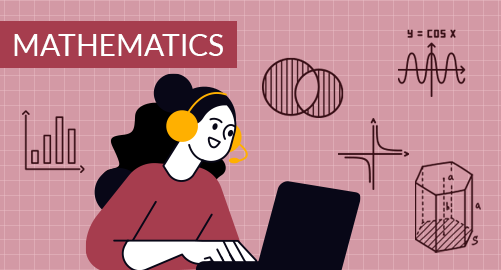#### through a demo today# Year 10 Mathematics

Mathematics
Year 10

The Year 10 Mathematics curriculum equips students with various skills that are essential for mathematical development and applying mathematical concepts to real-life situations. It covers topics from surds, algebra, polynomials, graphs, indices, logarithms, money and financial mathematics, area and volume, geometry, statistics, probability, trigonometry, and functions.

## What you'll learn

• 1

Revision of Numbers

• 2

Algebra

• 3

Financial Mathematics

• 4

Geometry

• 5

Coordinate Geometry

• 6

Measurement

• 7

Trigonometry

• 8

Data Analysis

• 9

Probability

• 10

Functional Operations

## Skills you'll learnNumber Analogy - The detail of surds and arithmetic operations on surds and simplification, rationalising factor, exponents, growth, and decay, and working with logarithms.Algebraic Proficiency Skills - Solving brackets, simplification of expressions, working with linear equations and inequalities, dealing with polynomial operations, and factorisation techniques.Money and Financial Skills - Understanding ratio and proportion, direct and inverse proportion, working with percentages, finding discounts, calculating discount and GST, percentage increase and decrease, simple and compound interest, and depreciation.Geometrical Skills and Spatial Reasoning - Extending thinking of similarity and congruence, enlargements, similarity within triangles and in circles, parts of circles, concept of tangent and secant, proving by theorems.Coordinate Geometry Competencies - Locating coordinates, understanding and finding regions of the plane, calculating cartesian equation, the graph of parabola and hyperbola, and understanding the intersection of graphs.Measurement Proficiency and Conversion Skills - Review of shapes, area, and volume of 3D shape measurement, unit conversions, and enlargements.Critical Thinking Skills - Applying Pythagoras theorem, using trigonometric ratios, understanding 3-D trigonometry, finding the area of triangles, and calculating trigonometric ratios for obtuse angles.Data Analysis and Interpretation - Data Organization, finding the central value of data, statistical data investigation process, sketching box plots, histograms and finding outliers, understanding deviation methods, working with time-series, and bivariate data.Prediction Skills - Understanding probability and related terms, event and outcome analysis, set operations in probability, estimating probability, handling multiple events, and sampling with replacement.Analytical Thinking - Terms of functions, range, domain, notations and working with functions, calculating inverse functions, understanding graph of functions, composite of inverse functions.